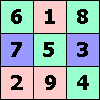ONE TO NINE by Andrew HodgesPage 44: There is a great deal in the Blood of the Isles about what can be learnt from Y-chromosomes and mitochondrial DNA about the population of the British Isles.

Page 40: Define (A NOR B) to mean 'neither A nor B is true'. Then A NOR A means the same as NOT A.
Then (A NOR A) NOR (B NOR B) means 'neither A nor B is false', which is the same as (A AND B).
(A NOR B) NOR (A NOR B) means 'It is not true that neither A nor B is true', which is the same as (A OR B).

Page 58: (0, 2) has the property (0, 2) × (0, 2) = (0 × 0 − 2 × 2, 0 × 2 + 0 × 2) = (−4, 0). So it is a square root of (−4). So is (0, −2).

You are unlikely to find a square root of (0, 1) by guessing. It needs a little algebra. Suppose it is (a, b).
Then we must have (a, b) × (a, b) = (0, 1), i.e. (a² − b², a × b + a × b) = (0, 1)
i.e. a² = b² and a × b = 1/2
So a = b and a² = 1/2,
so a = b = 1/√2, or a = b = − 1/√2.